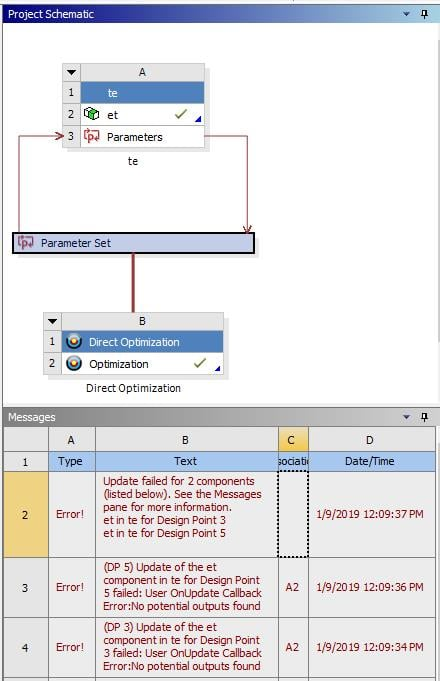# Errors about integrate executable into ANSYS Workbench using ACT

Member Posts: 3

I am working on integrating a Matlab script into Workbench using ACT. I first generate an executable file using Matlab and then using ACT to integrate it into Workbench. Everything goes well except some design points cannot be updated correctly. The project schematic and Messages are shown below, and the Matlab script is a simple one also shown below.Matlab Script:

function [x,y] = test(a,b)

x = a+b;

y = b*a;

end